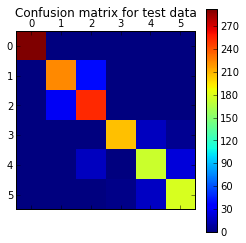## Results¶

### Training¶

We now run our RandomForest modeling software on our training set, described earlier, and derive a model along with some parameters describing how good our model is.

In :
%pylab inline
# We pull in the training, validation and test sets created according to the scheme described
# in the data exploration lesson.

import pandas as pd

# We use the Python RandomForest package from the scikits.learn collection of algorithms.
# The package is called sklearn.ensemble.RandomForestClassifier

# For this we need to convert the target column ('activity') to integer values
# because the Python RandomForest package requires that.
# In R it would have been a "factor" type and R would have used that for classification.

# We map activity to an integer according to
# laying = 1, sitting = 2, standing = 3, walk = 4, walkup = 5, walkdown = 6
# Code is in supporting library randomforest.py

import randomforests as rf
samtrain = rf.remap_col(samtrain,'activity')
samval = rf.remap_col(samval,'activity')
samtest = rf.remap_col(samtest,'activity')

In :
import sklearn.ensemble as sk
rfc = sk.RandomForestClassifier(n_estimators=500, compute_importances=True, oob_score=True)
train_data = samtrain[samtrain.columns[1:-2]]
train_truth = samtrain['activity']
model = rfc.fit(train_data, train_truth)

In :
# use the OOB (out of band) score which is an estimate of accuracy of our model.
rfc.oob_score_

Out:
0.97946768060836498
In :
### TRY THIS
# use "feature importance" scores to see what the top 10 important features are
fi = enumerate(rfc.feature_importances_)
cols = samtrain.columns
[(value,cols[i]) for (i,value) in fi if value > 0.04]
## Change the value 0.04 which we picked empirically to give us 10 variables
## try running this code after changing the value up and down so you get more or less variables
## do you see how this might be useful in refining the model?
## Here is the code in case you mess up the line above
## [(value,cols[i]) for (i,value) in fi if value > 0.04]

Out:
[(0.052194982088894198, 'tAccMean'),
(0.046418448022626055, 'tAccStd'),
(0.043291948466911298, 'tJerkMean'),
(0.053130159100753124, 'tGyroJerkMagSD'),
(0.059232069484007693, 'fAccMean'),
(0.048256742613275803, 'fJerkSD'),
(0.13799007369608407, 'angleGyroJerkGravity'),
(0.17036595812582825, 'angleXGravity'),
(0.044817236984266123, 'angleYGravity')]

We use the predict() function using our model on our validation set and our test set and get the following results from our analysis of errors in the predictions.

In :
# pandas data frame adds a spurious unknown column in 0 position hence starting at col 1
# not using subject column, activity ie target is in last columns hence -2 i.e dropping last 2 cols

val_data = samval[samval.columns[1:-2]]
val_truth = samval['activity']
val_pred = rfc.predict(val_data)

test_data = samtest[samtest.columns[1:-2]]
test_truth = samtest['activity']
test_pred = rfc.predict(test_data)


#### Prediction Errors and Computed Error Measures¶

In :
print("mean accuracy score for validation set = %f" %(rfc.score(val_data, val_truth)))
print("mean accuracy score for test set = %f" %(rfc.score(test_data, test_truth)))

mean accuracy score for validation set = 0.846477
mean accuracy score for test set = 0.895623

In :
# use the confusion matrix to see how observations were misclassified as other activities
# See 
import sklearn.metrics as skm
test_cm = skm.confusion_matrix(test_truth,test_pred)

In :
# visualize the confusion matrix

In :
import pylab as pl
pl.matshow(test_cm)
pl.title('Confusion matrix for test data')
pl.colorbar()
pl.show()In :
# compute a number of other common measures of prediction goodness


We now compute some commonly used measures of prediction "goodness".
For more detail on these measures see ,,,

In :
# Accuracy
print("Accuracy = %f" %(skm.accuracy_score(test_truth,test_pred)))

Accuracy = 0.895623

In :
# Precision
print("Precision = %f" %(skm.precision_score(test_truth,test_pred)))

Precision = 0.897903

In :
# Recall
print("Recall = %f" %(skm.recall_score(test_truth,test_pred)))

Recall = 0.895623

In :
# F1 Score
print("F1 score = %f" %(skm.f1_score(test_truth,test_pred)))

F1 score = 0.896047


### Exercise¶

Instead of using domain knowledge to reduce variables, use Random Forests directly on the full set of columns. Then use variable importance and sort the variables.

Compare the model you get with the model you got from using domain knowledge.
You can short circuit the data cleanup process as well by simply renaming the variables x1, x2...xn, y where y is 'activity' the dependent variable.

Now look at the new Random Forest model you get. It is likely to be more accurate at prediction than the one we have above. It is a black box model, where there is no meaning attached to the variables.

• What insights does it give you?
• Which model do you prefer?
• Why?
• Is this an absolute preference or might it change?
• What might cause it to change?
In :
from IPython.core.display import HTML
def css_styling():